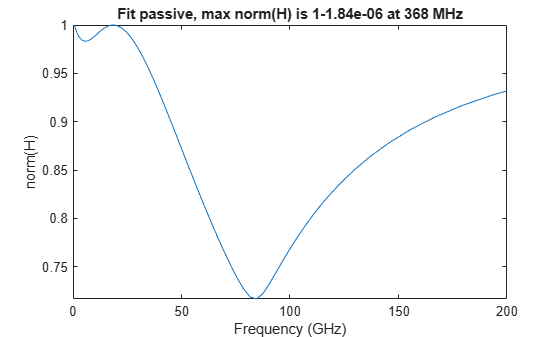Documentation

# makepassive

Enforce passivity of a rationalfit output

## Syntax

``pfit = makepassive(fit,s)``
``pfit = makepassive(fit,s,'Display','on')``

## Description

example

````pfit = makepassive(fit,s)` produces a passive fit by modifying the input, `fit` while optimally matching the data of S-parameter input, `s`. `makepassive` function does modifies the residues of the `fit` to make it passive.```
````pfit = makepassive(fit,s,'Display','on')` solves as above, but turns on the display of iteration information. The default for `'Display'` is `'off'`.```

## Examples

collapse all

Read a file named `passive.s2p` and fit the 2x2 S-parameters.

``` S = sparameters('passive.s2p'); fit = rationalfit(S);```

Plot the passivity of the 2x2 fit, noting the violations.

``` figure passivity(fit)```Optimize residues to produce a passive fit still close to S.

` pfit = makepassive(fit,S)`
```pfit=2×2 object 2x2 rfmodel.rational array with properties: A C D Delay Name ```

To display iteration information:

` pfit = makepassive(fit,S,'Display','on' )`
```ITER H-INFTY NORM FREQUENCY ERRDB CONSTRAINTS 0 1 + 1.791e-02 17.6816 GHz -40.4702 1 1 + 2.878e-04 275.327 MHz -40.9167 5 2 1 + 9.233e-05 365.865 MHz -40.9092 7 3 1 - 3.541e-07 368.137 MHz -40.906 9 ```
```pfit=2×2 object 2x2 rfmodel.rational array with properties: A C D Delay Name ```

Plot the passivity of the new fit.

``` figure passivity(pfit)```## Input Arguments

collapse all

N-by-N array, specified `rfmodel.rational` objects returned by `rationalfit`.

S-parameters, specified as an RF Toolbox™ network parameter object. To create this type of object, use the `sparameters` function.

## Output Arguments

collapse all

`rfmodel.rational` objects, returned as N-by-N array.